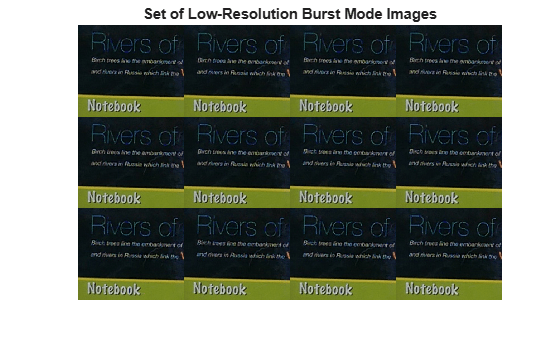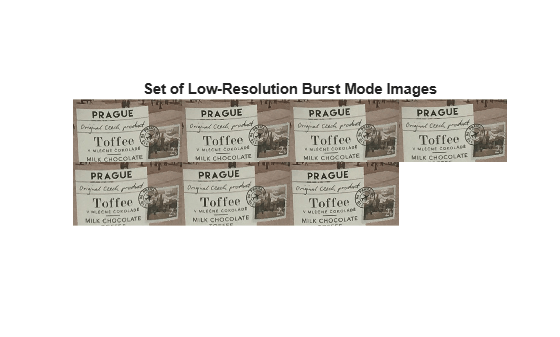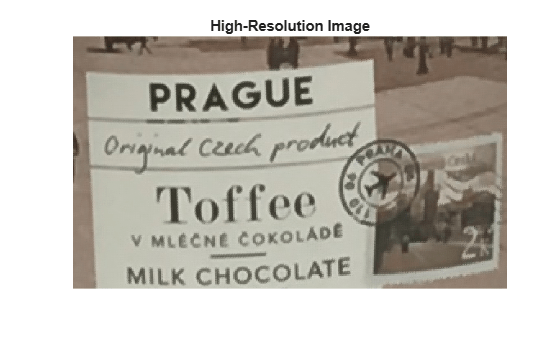# burstinterpolant

Create high-resolution image from set of low-resolution burst mode images

## Syntax

``B = burstinterpolant(imds,tforms,scale)``
``B = burstinterpolant(images,tforms,scale)``

## Description

example

````B = burstinterpolant(imds,tforms,scale)` creates a high-resolution image, `B` from a set of low-resolution burst mode images stored as an `ImageDatastore` object, `imds`. `scale` specifies the magnification value for high-resolution image. The size of `B` is `scale` times the size of input images.```

example

````B = burstinterpolant(images,tforms,scale)` creates a high-resolution image, `B` from a set of low-resolution burst mode images stored in cell array `images`. The size of `B` is `scale` times the size of input images.```

## Examples

collapse all

Specify the location of low-resolution burst mode images to be stored as an image datastore object. The input images are 2-D RGB images.

`setDir = fullfile(toolboxdir('images'),'imdata','notebook');`

Use the `imageDatastore` function to read and store the low-resolution burst mode images as an image datastore object.

`imds = imageDatastore(setDir,'FileExtensions',{'.png'});`

Display the images as a montage.

```montage(imds) title('Set of Low-Resolution Burst Mode Images')```Compute Geometric Transformation Parameters

To compute geometric transformation parameters, convert all the RGB images into lightness images by using `rgb2lightness` function. The burst mode lightness images are stored as an image datastore object.

`imdsTransformed = transform(imds,@(x) rgb2lightness(x));`

Read the first lightness image into the workspace and use it as the reference image for estimating geometric transformations.

`refImg = read(imdsTransformed);`

Get the optimal configuration parameters required for registration of the burst mode lightness images by using `imregconfig` function. Specify the image capture modality as `'monomodal'`.

`[optimizer,metric] = imregconfig('monomodal');`

Find the total number of images stored in the image datastore object by using `numpartitions` function.

`numImages = numpartitions(imds);`

Create an array of 2-D affine transformation object to store 2-D affine transformations of each low-resolution burst mode lightness image excluding the reference image. Set the number of rows in the transformation array as total number of images in the image datastore object minus one.

`tforms = repmat(affine2d(),numImages-1,1);`

Use the `imregtform` function to estimate the rigid geometric transformations for each low-resolution burst mode lightness image with respect to the reference image.

```idx = 1; while hasdata(imdsTransformed) movingImg = read(imdsTransformed); tforms(idx) = imregtform(refImg,movingImg,'rigid',optimizer,metric); idx = idx + 1; end```

Construct High-Resolution Image

Specify the scale factor for generating the high-resolution image.

`scale = 4;`

Create the high-resolution image from the set of low-resolution burst mode RGB images. Specify the transformation parameter to robustly estimate the high-resolution pixel values.

`B = burstinterpolant(imds,tforms,scale);`

Display the high-resolution image.

```figure('WindowState','maximized') imshow(B) title ('High-Resolution Image')```Read a low-resolution burst mode RGB image from the image datastore and display its size.

```Img = read(imds); inputDim = [size(Img,1) size(Img,2)]```
```inputDim = 1×2 161 186 ```

Display the size of the high-resolution image. Because the scale factor is 4, the size of the high-resolution image is 4 times the size of the low-resolution burst mode RGB images.

`outputDim = [size(B,1) size(B,2)]`
```outputDim = 1×2 644 744 ```

Load cell array data containing the low-resolution burst mode image into the workspace. The input images are monomodal and 2-D RGB images.

`load('LRData')`

Display images in the cell array data as a montage.

```montage(images,'Size',[2 4],'BackgroundColor',[1 1 1]); title('Set of Low-Resolution Burst Mode Images')```Compute Geometric Transformation Parameters

To compute geometric transformation parameters, convert all the RGB images into lightness images by using `rgb2lightness` function.

`imagesT = cellfun(@rgb2lightness,images,'UniformOutput',false);`

Read the first lightness image into the workspace and use it as the reference image for estimating geometric transformations.

`refImg = imagesT{1};`

Get the optimal configuration parameters required for registration of the burst mode lightness images by using `imregconfig`. Specify the image capture modality as `'monomodal'`.

`[optimizer,metric] = imregconfig('monomodal');`

Find the total number of images stored in the cell array.

`numImages = length(images);`

Create an array of 2-D affine transformation object to store 2-D affine transformations of each low-resolution burst mode lightness image excluding the reference image. Set the number of rows in the transformation array as total number of images in the cell array minus one.

`tforms = repmat(affine2d(),numImages-1,1);`

Use the `imregtform` function to estimate the rigid geometric transformations for each low-resolution burst mode lightness image with respect to the reference image.

```for i= 2:length(images) movingImg = imagesT{i}; tforms(i-1) = imregtform(refImg,movingImg,'rigid',optimizer,metric); end```

Construct High-Resolution Image

Specify the scale factor for generating the high-resolution image.

`scale = 3;`

Construct the high-resolution image from the set of low-resolution burst mode RGB images. Specify the transformation parameter to robustly estimate the high-resolution pixel values.

`B = burstinterpolant(images,tforms,scale);`

Display the high-resolution image.

```figure imshow(B); title ('High-Resolution Image')```Read a low-resolution burst mode RGB image from the cell array and display its size.

```Img = images{1}; inputDim = [size(Img,1) size(Img,2)]```
```inputDim = 1×2 154 265 ```

Display the size of the high-resolution image. Because the scale factor is 3, the size of the high-resolution image is 3 times the size of the low-resolution burst mode images.

`ouputDim = [size(B,1) size(B,2)]`
```ouputDim = 1×2 462 795 ```

## Input Arguments

collapse all

Input image datastore, specified as an `ImageDatastore` object. The input image datastore contains multiple low-resolution burst mode images used for creating the high-resolution image output.

• Images in the input image datastore must be 2-D grayscale images of size m-by-n or 2-D RGB images of size m-by-n-by-3.

• All images in the input image datastore must be of the same size and data type.

• The number of images in the input image datastore must be greater than or equal to 2.

Data Types: `single` | `double` | `uint8` | `uint16`

Input images, specified as a k-by-1 cell array. `k` is the number of input images stored in the cell array. All the input images must have same size.

Data Types: `single` | `double` | `uint8` | `uint16`

Transformation parameter, specified as an `affine2d` object array of size (k-1)-by-1 or 1-by-(k-1). k is the number of images in input `imds` or `images`.

Resize factor, specified as a scalar greater than or equal to 1.

Data Types: `single` | `double` | `int8` | `int16` | `int32` | `int64` | `uint8` | `uint16` | `uint32` | `uint64`

## Output Arguments

collapse all

High-resolution image, returned as a 2-D grayscale image or 2-D RGB image. `B` is of the same data type as the input images. The size of `B` is the value of `scale` times the size of the images in input `imds` or `images`.

For example, let L be the value of `scale`, and m-by-n be the size of the low-resolution burst mode images. Then, the size of the high-resolution image is mL-by-nL.

## Algorithms

The `burstinterpolant` function uses the inverse distance weighting method  to generate high-resolution image from a set of low-resolution burst mode images. The function predicts a high-resolution pixel value from a set of pixels in the low-resolution burst mode images, selected based on the transformation parameter. The use of transformation parameter `tforms` makes the pixel selection robust to any rigid geometric transformations (rotations and translations only).

### Note

• If the input images are 2-D RGB images, estimate `tforms` from the lightness component. You can use the `rgb2lightness` function to compute lightness values from the RGB color values.

 Shepard, Donald. “A Two-Dimensional Interpolation Function for Irregularly-Spaced Data”, In Proceedings of the 1968 23rd ACM National Conference, 517-524. New York, NY: ACM, 1968.